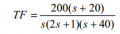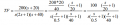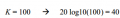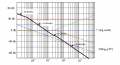# Bode Plot analysis and drawing in semi log graph

Thread Starter

#### mishra87

Joined Jan 17, 2016
906
Dear All,

Hope all is well.

I am trying to understand the bode plot in semi log graph of below transfer function.The simplify transfer function form:Constant factor:db

1 pole at the origin :
1 zero at z1 = 20
2 poles: at p1 = 0.5 and p2=40

Now its very difficult for me understand bode Gain and Phase plot :Could anybody make me understand how to draw above plot of Gain and Phase and how to calculate Gain and Phase Margin.
Please go step by step so it would be easy to understand.
Lets say-
Why bold black line statred from 60db ???
Where is pole and zero added and what is what changes in db/decade.???
How do we calculate db/decade changes ???

Please consider me as a novice.

Thanks !!!

Last edited:

#### drc_567

Joined Dec 29, 2008
1,114
The 60 db gain at the left margin is the 40 db constant gain, plus an extrapolation of the single simple pole, with slope -20 db per decade, going through the 0 dB gain point on the abscissa, where frequency equals 1 ... (10^0).

Last edited:

#### drc_567

Joined Dec 29, 2008
1,114
Poles and zeroes with unity exponent have a diagram slope of -20 dB per decade for poles and +20 dB per decade for zeroes.

Root/zero factors with exponents equal to 2 have similar magnitude quantities equal to 40 db per decade. (Either + or - slope).

Basically, you can have either single root factors, or double root factors. Higher order factors, 3 and above, simply divide into the single and double root factors, if that makes sense. A quadratic factor can be two real roots, or two complex roots with oscillation ... have to review that one though.

Last edited:

#### drc_567

Joined Dec 29, 2008
1,114
The bold black line is simply the sum of the individual pole and zero factor contributions, as the frequency progresses.

#### drc_567

Joined Dec 29, 2008
1,114
If you examine the individual factors in the system transfer function, there is a specific break frequency associated with each pole or zero. Try to enumerate or define that frequency for each factor, and list all of them sequentially, including the pole at zero. This procedure should assist you in plotting the desired graph.

Thread Starter

#### mishra87

Joined Jan 17, 2016
906
Thank you so much drc_567 !!!

I stiil did not understood clearly could anybody elaborate a bit more !!!

Thanks !!!

Thread Starter

#### mishra87

Joined Jan 17, 2016
906
A expect a bit more explanation of above bode plot from this group.

Thanks !!!

Similar threads